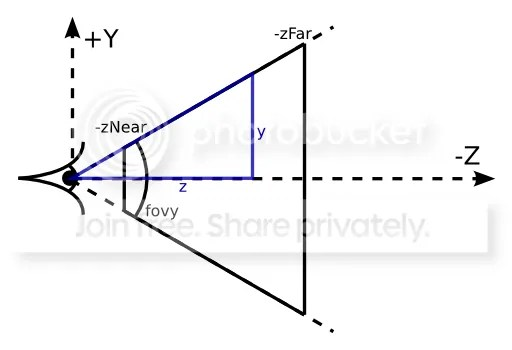For one of my projects, I drew asteroids coming toward you in space.

I started with the asteroids far away, and increased their z value (remembering it is negative!) to make them come closer.

I had two problems to solve

• when adding new asteroids at (say) a distance of z = -1000, I wanted to make them appear randomly all over the screen. How far from the middle can I place them without them being off the edge of the screen? In other words, how wide/high is the visible screen at a distance of 1000 (or any other distance)?
• as the asteroids come closer, they will nearly all disappear off one of the four sides of the screen before they reach the camera, and I want to stop drawing them when that happens. How do I know when that is?

This post explains the answers, and how perspective works in 3D.

I have never properly understood the perspective calculations, but this page helped me a lot, and I’m going to try to simplify it further for you, without any difficult math – and then answer the two questions above.

First, let’s figure out the height y of the visible screen, at a distance of z, as shown in the diagram below (taken from the web page I mentioned above).We want to calculate y. We know z (the distance from the camera), and we know the angle of the triangle, because when we give Codea the perspective command, we give it the size of the viewing angle. Actually, we usually don’t bother to do this, and Codea then uses 45 degrees as a default. So let’s assume the viewing angle is 45 degrees.

Half of that angle is above the line marked z, and half below, so the angle we are interested in, for the blue triangle, is half of 45, ie 22.5 degrees.

Using simple trigonometry, we know that y /- z = tan (22.5 degrees),

so y = -z  * tan(22.5 degrees) = 0.414 * -z

### Height and width of 3D screen

So if z = 1000, then y = 414, which means we can add asteroids anywhere between -414 and +414, for y.

You would expect to calculate x the same way and get the same answer, but remember that the screen is not square. The height is used as the baseline, and the x value has to be adjusted for the “aspect ratio” = screen width/ screen height.

So  x = 0.414 * -z * (WIDTH/HEIGHT)

If we are using the full Codea screen, with width of 1024 and height of 768, and a distance of 1000, then

x = 0.414 * 1000 * 1024 / 768 = 552.

So we add asteroids anywhere between -552 and +552, for x.

In fact, Codea does all the hard work for you, so you don’t even need to work any of this out for yourself. The projectionMatrix() has the x and y factors in it. They are inverted, ie they are 1 / the values above, so we need to divide them, as shown below.

```perspective()
camera(0,0,0,0,0,-1) --looking at -z
z = -1000
xMax = -z / projectionMatrix() --remember the round brackets
yMax = -z / projectionMatrix()
--set random position of new asteroid
--so the x value will be between -xMax and +xMax
pos = vec3(math.random(-xMax, xMax), math.random(-yMax, yMax), z)
```

### Figuring out when objects fall off the edges

This is quite easy, now we’ve figured out the height and width of the screen.

```perspective()
camera(0,0,0,0,0,-1) --looking at -z
--suppose we're looking at just one asteroid, positioned at (x,y,z)
xMax = -z / projectionMatrix()
yMax = -z / projectionMatrix()
if math.abs(x)>xMax or math.abs(y)>yMax then
--delete asteroid
end
```

The projectionMatrix factors will be constant, assuming you don’t change the perspective settings, so you can put them in variables as part of setup, and use them in the calculations, saving a little bit of looking up. If you do this, then note that you must give the perspective and camera commands first, to set up the projection matrix. You can do this in setup, like so.

```--in setup
perspective()
camera(0,0,0,0,0,-1) --looking at -z
xFactor = -1 / projectionMatrix()
yFactor = -1 / projectionMatrix()

--in draw, when checking if an asteroid at (x,y,z) is still on screen
if math.abs(x)> z * xFactor or math.abs(y)> z * yFactor then
--delete asteroid
end
```

### There’s just one problem

All my calculations above are based on the centre point of the asteroid. But asteroids are not single points, but 3D, so the code above will draw some asteroids that don’t fit on the screen fully, and remove others when they are still partly on the screen.

It’s easy to adjust for the size of an asteroid. When adding them, simply reduce the width/height of the screen by the radius, to make sure they fit on the screen fully. When testing if they’ve fallen off the screen, reduce the absolute values of x and y by the radius, before testing them. So, in the last example above, we would have, for radius r,

```if math.abs(x)-r> z * xFactor or math.abs(y)-r> z * yFactor then
```

### Example

The video below shows the code working in practice. To prove that the asteroids are being created and deleted correctly, I adjusted the screen width and height factors to 70% of their normal size, as shown by the rectangle. You should see all asteroids are created within the rectangle, and as they move outside it, they disappear.

The code is here.